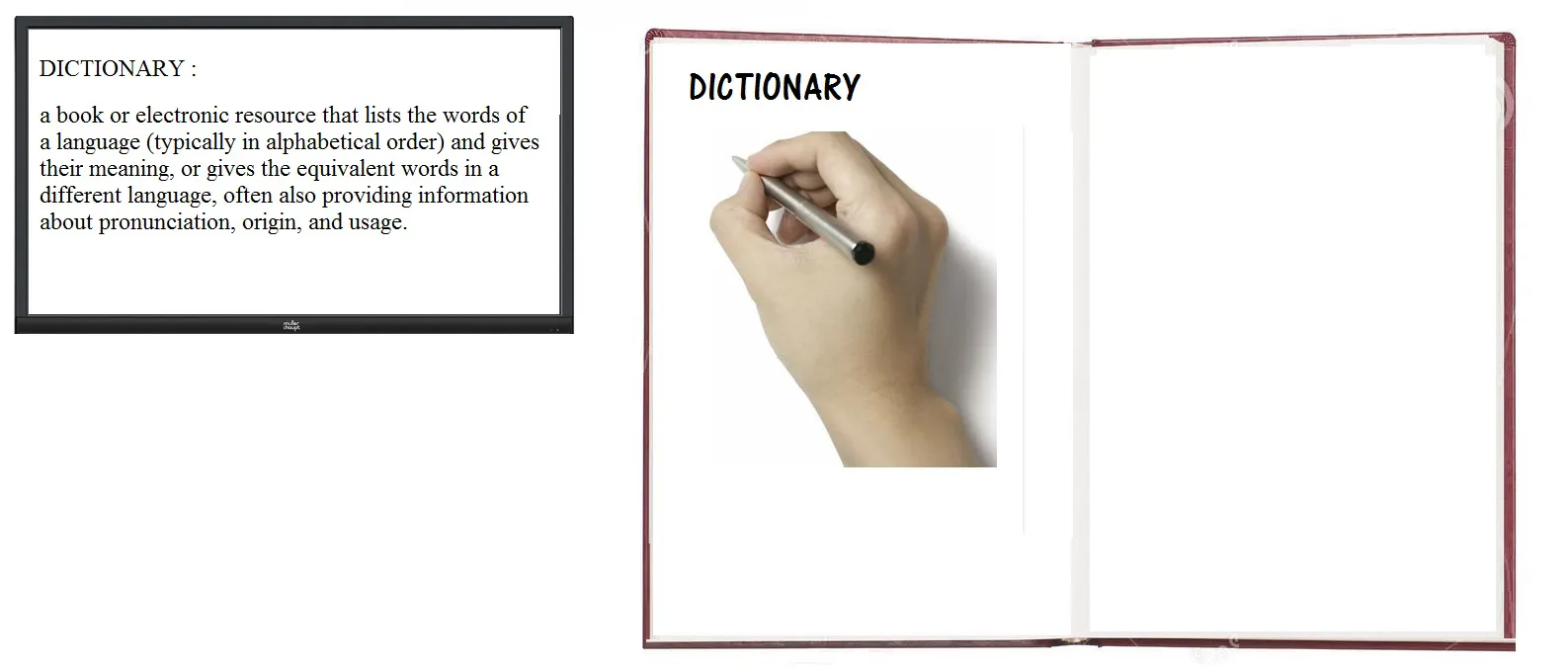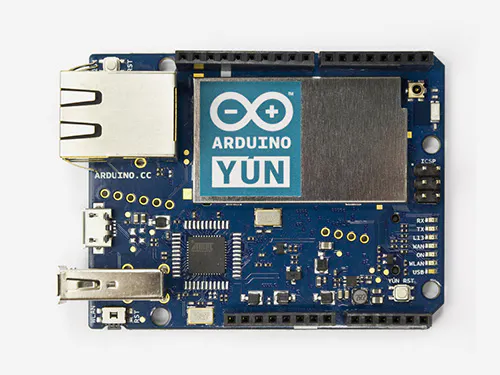Created January 29, 2016

# Smart Dictionary

A pen can teach you the meaning of a word that you write with the pen.

IntermediateShowcase (no instructions)564## Things used in this project

### Hardware componentsAmazon Web Services AWS CloudWatch
×1Arduino Yun
×1

## Code

### Untitled file

Arduino
```// include the SPI library:
#include <SPI.h>
#include<alphabetscode.h>
// setup pins
int nReset = 12;
int nCS = 13;
int m,mn,m',mn';
int ContactSensor= 5;
int x[200,26],y[200,26];
\\x and y are the the respective coordinates of each letter stored as an array:
\\Enable refers to the end of the word:
\\t is the serial number of letters and i is an iterating variable:
int i,t;
int Enable= A0;
void setup(){
//set pin I/O direction
pinMode (nReset, OUTPUT);
pinMode (5, INPUT);
pinMode (A0, INPUT);
pinMode (nCS, OUTPUT);
//put the device in reset not chip selected
digitalWrite(nReset,LOW);
digitalWrite(nCS,HIGH);
//initialize SPI
SPI.begin();
SPI.setDataMode(SPI_MODE3);
//start a serial port for debugging
Serial.begin(115200);
delay(1000);
Serial.println("startup complete");
}
void loop(){
delay(1000);
digitalWrite(nReset,HIGH);
//startup time
delay(1000);
//read and print a register map
delay(1000);
}
void ADNS_write(unsigned int address, unsigned int data){
// take the CS pin low to select the chip:
digitalWrite(nCS,LOW);
//  send in the address and value via SPI:
SPI.transfer(data);
// take the SS pin high to de-select the chip:
digitalWrite(nCS,HIGH);
}
{
unsigned int data;
// take the CS pin low to select the chip:
digitalWrite(nCS,LOW);
//  send in the address and value via SPI:
pinMode(MOSI, INPUT);
data = SPI.transfer(0x00);
pinMode(MOSI, OUTPUT);
// take the SS pin high to de-select the chip:
digitalWrite(nCS,HIGH);
return(data);
x[0,t]=0;
y[0,t]=0;
t=0;
\\While(Enable) to denote the end of the word and contact sensor to determint whether the pen is writing:
while(Enable=0)
{
if(ContactSensor>0)
{
t=t+1;
//there has been a motion!
//print the x and y movements
{
for ( i = 0; i < 200; i++ )
{
}
}
\\After writing a letter the contact sensor is 0 till the next letter is written for text in CAPS:
\\Text processing and storing the previous letter:
if(ContactSensor=0)
{
if(t>=1))
m = max(x[i,t-1]);
mn = min(x[i,t-1]);
m' = max(y[i,t-1]);
mn' = min(y[i,t-1]);
x'[i,t-1] = map(x[i,t-1], mn, m, 0,10);
y'[i,t-1] = map(y[i,t-1], mn', m', 0,10);
\\The letters in my own header file(alphabetscode.h) that matches with the coordinates of the alphabet of the written word:
\\alphabetscode.max() is to find the letters coordinates that best matches with the library:
alphabetscode.max()
{
println("The alphabet is" aplhabetscode.max())
}
\\Adding the word letter by letter to a file - input.txt:
for ( i = 0; i < 200; i++ )
{
Serial.println(x'[i,t-1],y'[i,t-1]);
Serial mySerial;
PrintWriter output;
\\Writing the value of arrays in a file named input:
void setup() {
mySerial = new Serial( this, Serial.list(), 9600 );
output = createWriter( "input.txt" );
}
void draw() {
if (mySerial.available() > 0 ) {
if ( x'[i,t-1]||y'[i,t-1] != null )
{
output.println( x'[i,t-1],y'[i,t-1] );
}
if ( x'[i,t-1]||y'[i,t-1] = null )
{
output.println('\n');
\\To denote the end of the letter in the file:
}

}
}
}
\\The letter is added to the file and closed:
while(Enable>0)
void keyPressed()
{
output.flush();  // Writes the remaining data to the file
output.close();  // Finishes the file
exit();  // Stops the program
}
\\When Enable is 1 the file is closed:
}

}
```

## Credits

### Kapildev Kumar

3 projects • 8 followers
Always cool. Addicted to Work.